# Ohio Open Ed Collaborative Pre-Calculus

This content was created as part of an Ohio Department of Higher Education Innovation Grant to create Open Educational Resources for high enrollment courses. A team of faculty content collaborators, a librarian, and a faculty review team worked together to curate this content and assure that it meets the Transfer Assurance Guidelines for this course. The Pre-Calculus Course Content is designed to help the instructor teach all of the objectives of the course and can be used as a whole or in pieces or modules. The full course is entitled Pre-Calculus Course Content. This work was completed and the courses were posted in September 2019. Please visit ohioopened.org for more information about this initiative.

61 affiliated resources

# Search Resources

View
Selected filters:Rating

The Pre-Calculus course was developed through the Ohio Department of Higher Education OER Innovation Grant. This work was completed and the course was posted in September 2019. The course is part of the Ohio Transfer Module and is also named TMM002. For more information about credit transfer between Ohio colleges and universities, please visit: www.ohiohighered.org/transfer.Team LeadKameswarrao Casukhela                   Ohio State University LimaContent ContributorsLuiz Felipe Martins                             Cleveland State UniversityIeda Rodrigues                                   Cleveland State UniversityTeri Thomas                                        Stark State CollegeLibrarianDaniel Dotson                                     Ohio State University                     Review TeamAlice Taylor                                        University of Rio GrandeRita Ralph                                          Columbus State Community College

Subject:
Applied Science
Education
Mathematics
Calculus
Physical Science
Material Type:
Full Course
Provider:
Ohio Open Ed Collaborative
01/09/2019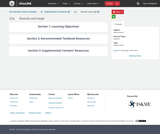Conditions of Use:
Remix and Share
Rating

Sine and Cosine Functions - amplitude, period, phase-shift, sinusoidal functions, periodic functionsTMM 002 PRECALCULUS (Revised March 21, 2017)1. Functions: 1a. Analyze functions. Routine analysis includes discussion of domain, range, zeros, general function behavior (increasing, decreasing, extrema, etc.), as well as periodic characteristics such as period, frequency, phase shift, and amplitude. In addition to performing rote processes, the student can articulate reasons for choosing a particular process, recognize function families and anticipate behavior, and explain the implementation of a process (e.g., why certain real numbers are excluded from the domain of a given function).*

Subject:
Mathematics
Calculus
Trigonometry
Material Type:
Module
Provider:
Ohio Open Ed Collaborative
05/28/2019Conditions of Use:
Remix and Share
Rating

Secant and Cosecant Functions - period, phase-shift, periodic functions, asymptotes, sine function, cosine function, domainTMM 002 PRECALCULUS (Revised March 21, 2017)1. Functions: 1a. Analyze functions. Routine analysis includes discussion of domain, range, zeros, general function behavior (increasing, decreasing, extrema, etc.), as well as periodic characteristics such as period, frequency, phase shift, and amplitude. In addition to performing rote processes, the student can articulate reasons for choosing a particular process, recognize function families and anticipate behavior, and explain the implementation of a process (e.g., why certain real numbers are excluded from the domain of a given function).*

Subject:
Mathematics
Calculus
Trigonometry
Material Type:
Module
Provider:
Ohio Open Ed Collaborative
05/28/2019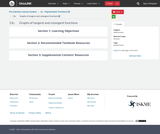Conditions of Use:
Remix and Share
Rating

Tangent and Cotangent Functions - period, phase-shift, periodic functions, asymptotes, sine and cosine functionsTMM 002 PRECALCULUS (Revised March 21, 2017)1. Functions: 1a. Analyze functions. Routine analysis includes discussion of domain, range, zeros, general function behavior (increasing, decreasing, extrema, etc.), as well as periodic characteristics such as period, frequency, phase shift, and amplitude. In addition to performing rote processes, the student can articulate reasons for choosing a particular process, recognize function families and anticipate behavior, and explain the implementation of a process (e.g., why certain real numbers are excluded from the domain of a given function).*

Subject:
Higher Education
Mathematics
Calculus
Trigonometry
Material Type:
Module
Provider:
Ohio Open Ed Collaborative
05/28/2019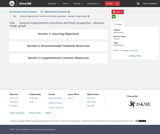Conditions of Use:
Remix and Share
Rating

Inverse Trigonometric Functions - domain, range, graph, one-to-one function, applications, periodic functions TMM 002 PRECALCULUS (Revised March 21, 2017)1. Functions: 1a. Analyze functions. Routine analysis includes discussion of domain, range, zeros, general function behavior (increasing, decreasing, extrema, etc.), as well as periodic characteristics such as period, frequency, phase shift, and amplitude. In addition to performing rote processes, the student can articulate reasons for choosing a particular process, recognize function families and anticipate behavior, and explain the implementation of a process (e.g., why certain real numbers are excluded from the domain of a given function).*

Subject:
Mathematics
Calculus
Trigonometry
Material Type:
Module
Provider:
Ohio Open Ed Collaborative
05/28/2019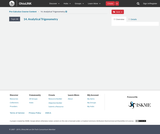Conditions of Use:
Remix and Share
Rating

Sinusoidal function, harmonic motion, periodic functions, applications.TMM 002 PRECALCULUS (Revised March 21, 2017)1. Functions: 1a. Analyze functions. Routine analysis includes discussion of domain, range, zeros, general function behavior (increasing, decreasing, extrema, etc.), as well as periodic characteristics such as period, frequency, phase shift, and amplitude. In addition to performing rote processes, the student can articulate reasons for choosing a particular process, recognize function families and anticipate behavior, and explain the implementation of a process (e.g., why certain real numbers are excluded from the domain of a given function).*

Subject:
Mathematics
Calculus
Trigonometry
Physics
Material Type:
Module
Provider:
Ohio Open Ed Collaborative
05/28/2019Conditions of Use:
Remix and Share
Rating

Law of CosinesTMM 002 PRECALCULUS (Revised March 21, 2017)2c. Analyze general triangles. Routine analysis of side lengths and angle measurements using trigonometric ratios/functions, as well as other relationships.*Sample Tasks:The student can solve general triangles using trigonometric ratios and relationships including laws of sine and cosine.The student can compare similar triangles.The student can compute length and angle measurements inside complex drawings involving multiple geometric objects.The student can algebraically describe relationships inside complex drawings involving multiple geometric objects.

Subject:
Mathematics
Calculus
Geometry
Trigonometry
Material Type:
Module
Provider:
Ohio Open Ed Collaborative
05/28/2019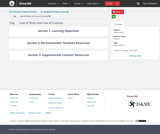Conditions of Use:
Remix and Share
Rating

Law of SinesTMM 002 PRECALCULUS (Revised March 21, 2017)2c. Analyze general triangles. Routine analysis of side lengths and angle measurements using trigonometric ratios/functions, as well as other relationships.*Sample Tasks:The student can solve general triangles using trigonometric ratios and relationships including laws of sine and cosine.The student can compare similar triangles.The student can compute length and angle measurements inside complex drawings involving multiple geometric objects.The student can algebraically describe relationships inside complex drawings involving multiple geometric objects.

Subject:
Higher Education
Mathematics
Calculus
Geometry
Trigonometry
Material Type:
Module
Provider:
Ohio Open Ed Collaborative
05/28/2019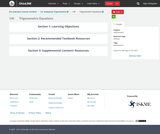Conditions of Use:
Remix and Share
Rating

Trigonometric Equations, trigonometric identitiesTMM 002 PRECALCULUS (Revised March 21, 2017)4c. Become fluent with conversions using traditional equivalency families.*(e.g., (sin(𝑡))2+(cos(𝑡))2=1; (tan(𝑡))2+1=(sec(𝑡))2; sums/differences; products; double angle; Euler’s Formula (𝑒𝑖𝜃=cos(𝜃)+𝑖sin(𝜃)); etc.)Sample Tasks:The student can prove trigonometric identities.The student solves trigonometric equations.To solve √cos(4𝑡) = √sin(4𝑡), the student solves cos(4𝑡) =sin(4𝑡) and knows this procedure may result in extraneous solutions.The student solves |cos (2𝜃−3)| + 32 = 2 by rewriting the left-hand side as a piecewise-defined function.The student can rewrite formulas involving multiple occurrences of the variable to formulas involving a single occurrence. Write 𝑎sin(𝑤 𝑡)+𝑏cos(𝑤 𝑡) as 𝐴 sin (𝑤 𝑡+𝐵) or 𝐵 cos (𝑤 𝑡+𝐵). The student can rewrite sums as products to reveal attributes such as zeros, envelopes, and phase interference.The student can solve 2 𝑠𝑖𝑛2(𝑡)+7sin(𝑡)−4=0 on a given interval.The student can solve 𝑙𝑜𝑔4(sin (𝑡))+𝑙𝑜𝑔4(2sin(𝑡)+7)=1 on a given interval.

Subject:
Mathematics
Calculus
Trigonometry
Material Type:
Module
Provider:
Ohio Open Ed Collaborative
05/28/2019Conditions of Use:
Remix and Share
Rating

Complex numbers, Euler's notation, sine-cosine representation, DeMoivre's Theorem, product and quotient of complext numbers, powers and roots of complex numbers, magnitude, polar coordinates,

Subject:
Mathematics
Calculus
Trigonometry
Material Type:
Module
Provider:
Ohio Open Ed Collaborative
05/28/2019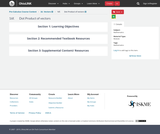Conditions of Use:
Remix and Share
Rating

Vectors - dot product, projection, decomposition of a vectorTMM 002 PRECALCULUS (Revised March 21, 2017)AdditionalOptional Learning Outcomes:2. Geometry: The successful Precalculus student can:2e. Interpret the result of vector computations geometrically and within the confines of a particular applied context (e.g., forces).Sample Tasks:The student can define vectors, their arithmetic, their representation, and interpretations.The student can decompose vectors into normal and parallel components.The student can interpret the result of a vector computation as a change in location in the plane or as the net force acting on an object.

Subject:
Engineering
Higher Education
Mathematics
Geometry
Trigonometry
Material Type:
Module
Provider:
Ohio Open Ed Collaborative
05/28/2019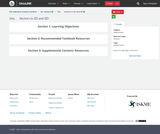Conditions of Use:
Remix and Share
Rating

Vectors - magnitude, direction, component form, trigonometric form, unit vector, algebra of vectors, applications,TMM 002 PRECALCULUS (Revised March 21, 2017)AdditionalOptional Learning Outcomes:2. Geometry: The successful Precalculus student can:2e. Interpret the result of vector computations geometrically and within the confines of a particular applied context (e.g., forces).Sample Tasks:The student can define vectors, their arithmetic, their representation, and interpretations.The student can decompose vectors into normal and parallel components.The student can interpret the result of a vector computation as a change in location in the plane or as the net force acting on an object.

Subject:
Higher Education
Mathematics
Calculus
Geometry
Physics
Material Type:
Module
Provider:
Ohio Open Ed Collaborative
05/28/2019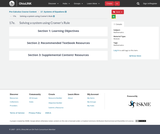Conditions of Use:
Remix and Share
Rating

TMM 002 PRECALCULUS (Revised March 21, 2017)3.  Equations and Inequalities:     3a.  Recognize function families as they appear in equations and inequalities and choose an appropriate solution methodology for a particular equation or inequality, as well as communicate reasons for that choice.*

Subject:
Mathematics
Material Type:
Module
Provider:
Ohio Open Ed Collaborative
05/28/2019Conditions of Use:
Remix and Share
Rating

TMM 002 PRECALCULUS (Revised March 21, 2017)1.  Functions:     1c.  Perform operations with functions including addition, subtraction, multiplication, division, composition, and inversion.*

Subject:
Mathematics
Material Type:
Module
Provider:
Ohio Open Ed Collaborative
05/28/2019Conditions of Use:
Remix and Share
Rating

TMM 002 PRECALCULUS (Revised March 21, 2017)3.  Equations and Inequalities:     3f.  Solve systems of equations using substitution and/or elimination.*

Subject:
Mathematics
Material Type:
Module
Provider:
Ohio Open Ed Collaborative
05/28/2019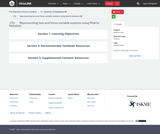Conditions of Use:
Remix and Share
Rating

TMM 002 PRECALCULUS (Revised March 21, 2017)3.  Equations and Inequalities:     3f.  Solve systems of equations using substitution and/or elimination.*

Subject:
Mathematics
Material Type:
Module
Provider:
Ohio Open Ed Collaborative
05/28/2019Conditions of Use:
Remix and Share
Rating

TMM 002 PRECALCULUS (Revised March 21, 2017)3.  Equations and Inequalities:     3a.  Recognize function families as they appear in equations and inequalities and choose an appropriate solution methodology for a particular equation or inequality, as well as communicate reasons for that choice.*

Subject:
Mathematics
Material Type:
Module
Provider:
Ohio Open Ed Collaborative
05/28/2019Conditions of Use:
Remix and Share
Rating

Ellipse - conic section, foci, major and minor axes, vertices, standard form, eccentricityTMM 002 PRECALCULUS (Revised March 21, 2017)AdditionalOptional Learning Outcomes:2. Geometry: The successful Precalculus student can:2f. Represent conic sections algebraically via equations of two variables and graphically by drawing curves.Sample Tasks:The student can perform the process “completing the square” transforming the equation into a standard form.The student can draw curves representing conic sections.The student can solve systems of equations involving linear and quadratic functions.The student can parametrize conic curves.

Subject:
Higher Education
Mathematics
Algebra
Geometry
Astronomy
Material Type:
Module
Provider:
Ohio Open Ed Collaborative
05/28/2019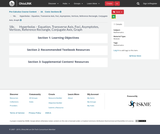Conditions of Use:
Remix and Share
Rating

Hyperbola - conic section, foci, transverse and conjugate axes, vertices, asymptotes, standard formTMM 002 PRECALCULUS (Revised March 21, 2017)AdditionalOptional Learning Outcomes:2. Geometry: The successful Precalculus student can:2f. Represent conic sections algebraically via equations of two variables and graphically by drawing curves.Sample Tasks:The student can perform the process “completing the square” transforming the equation into a standard form.The student can draw curves representing conic sections.The student can solve systems of equations involving linear and quadratic functions.The student can parametrize conic curves.

Subject:
Higher Education
Mathematics
Geometry
Astronomy
Material Type:
Module
Provider:
Ohio Open Ed Collaborative
05/28/2019Conditions of Use:
Remix and Share
Rating

Parabola - conic section, focus, directrix, focal length, standard form, vertex form, completing the square method.TMM 002 PRECALCULUS (Revised March 21, 2017)AdditionalOptional Learning Outcomes:2. Geometry: The successful Precalculus student can:2f. Represent conic sections algebraically via equations of two variables and graphically by drawing curves.Sample Tasks:The student can perform the process “completing the square” transforming the equation into a standard form.The student can draw curves representing conic sections.The student can solve systems of equations involving linear and quadratic functions.The student can parametrize conic curves.

Subject:
Higher Education
Mathematics
Algebra
Geometry
Material Type:
Module
Provider:
Ohio Open Ed Collaborative
05/28/2019Conditions of Use:
Remix and Share
Rating

Subject:
Mathematics
Material Type:
Module
Provider:
Ohio Open Ed Collaborative
05/21/2019Conditions of Use:
Remix and Share
Rating

Subject:
Mathematics
Material Type:
Module
Provider:
Ohio Open Ed Collaborative
05/21/2019Conditions of Use:
Remix and Share
Rating

Subject:
Mathematics
Material Type:
Module
Provider:
Ohio Open Ed Collaborative
05/21/2019Conditions of Use:
Remix and Share
Rating

Subject:
Mathematics
Material Type:
Module
Provider:
Ohio Open Ed Collaborative
05/21/2019Conditions of Use:
Remix and Share
Rating

Subject:
Mathematics
Material Type:
Module
Provider:
Ohio Open Ed Collaborative
05/28/2019Conditions of Use:
Remix and Share
Rating

Subject:
Mathematics
Material Type:
Module
Provider:
Ohio Open Ed Collaborative
05/28/2019Conditions of Use:
Remix and Share
Rating

Subject:
Mathematics
Material Type:
Module
Provider:
Ohio Open Ed Collaborative
05/28/2019Conditions of Use:
Remix and Share
Rating

Subject:
Mathematics
Material Type:
Module
Provider:
Ohio Open Ed Collaborative
05/28/2019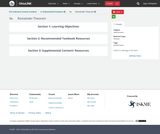Conditions of Use:
Remix and Share
Rating

Subject:
Mathematics
Material Type:
Module
Provider:
Ohio Open Ed Collaborative
05/28/2019Conditions of Use:
Remix and Share
Rating

Subject:
Mathematics
Material Type:
Module
Provider:
Ohio Open Ed Collaborative